﻿ Chapter 12: A Unified Approach to Option Pricingoffice (412) 9679367
fax (412) 967-5958
toll-free 1 (800) 214-3480

## CHAPTER 12:  A Unified Approach to Option Pricing

12.1  Equality of Volatility-Adjusted Drifts

 I

n this topic, we present a general method for valuing options which is particularly useful for valuing options on currencies and on futures, but also applies to common stock.  The method relies on the fact that if two assets are defined on the same source of uncertainty, then no arbitrage implies that their “volatility-adjusted excess drift” must be the same.  (Some expositions call this the market price of risk; we refrain from doing so because it confuses the drift rate with the expected return.)

The general principle is expressed in the following lemma, due to Garman (1976).

Lemma:

Let f and g be given by df = mfdt + sfdz and dg = ngdt + rgdz.  If r is the instantaneous risk-free interest rate, then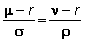We will show you why this provides a powerful method for valuing options after we show you the proof.  To prove the lemma, we

1.  construct a riskless portfolio using the two assets, and

2.  use the fact that this portfolio must yield the risk-free return.

For Step 1, consider the portfolio in which you are long a = (gr) units of f and short  b  = (fs) of g.  The value of this portfolio is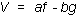The change in the portfolio over an instant of time is given by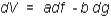Now, substitute for df and dg, and also for a and b, to get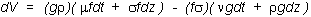Now, look at the two terms involving dz.  They are (gr)(sf)dz and -(fs)(rg)dz, which cancel.  Therefore, dV depends only on time, and is risk-free:We now use the fact that this portfolio must yield the risk-free return.  In other words,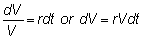Substitute for dV and for V = af - bg to get: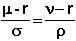You can see why we call each term the “volatility-adjusted excess drift.”

For the remaining part of this chapter we will apply this lemma to option valuation problems.  In the topic Application: Stock Options you will see how the basic Black-Scholes partial differential equation is derived, followed by applications to both currency options and options on futures.